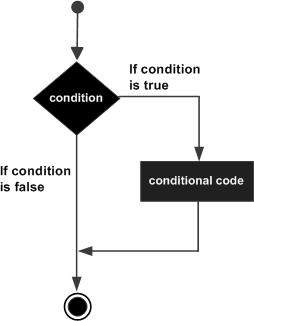# Dart Programming - If Statement

The if…else construct evaluates a condition before a block of code is executed.

Following is the syntax.

```if(boolean_expression){
// statement(s) will execute if the boolean expression is true.
}
```

If the Boolean expression evaluates to be true, then the block of code inside the if statement will be executed. If Boolean expression evaluates to be false, then the first set of code after the end of the if statement (after the closing curly brace) will be executed.

The following illustration shows the flowchart of the if statement.## Example

The following example shows how you can use the if statement in Dart.

```void main() {
var  num=5;
if (num>0) {
print("number is positive");
}
}
```

The above example will print “number is positive” as the condition specified by the if block is true.

```number is positive
```
dart_programming_decision_making.htm